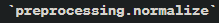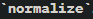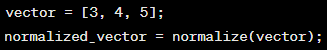# What is The Best Practice to Learn How to Normalize A Vector?

• Post published:June 22, 2023

Vector plays a significant role in the fields of mathematics, physics, computer science, and machine learning. The reason is that it represents quantities and directions to formulate an agile and dynamic structure. Normalizing a vector is a fundamental operation that scales a vector to have a magnitude of 1 while preserving its direction.

Learning the techniques and technologies associated with the normalization of the vector is crucial to be learned by a student, programmer, and data scientist. Keeping that in mind we have brought to you a detailed guide on the concept of how to normalize a vector while simultaneously mentioning the different strategies, and demonstration of implementations.

Besides, here in this article, you will also find details about some useful tools like NumPy and OpenGl that help in the smooth completion of this process.

## Understanding Vector Normalization Tools

Before diving into the techniques, let’s first clarify what vector normalization entails. A vector is typically represented as an array of numbers or coordinates in a multi-dimensional space. It consists of both magnitude (length) and direction.

Normalizing a vector involves dividing each component of the vector by its magnitude, resulting in a unit vector (a vector with a magnitude of 1). By doing so, we eliminate the influence of the vector’s original length and focus solely on its direction.

### L2 Normalization

One common technique for normalizing vectors is L2 normalization, also known as Euclidean normalization or unit vector normalization. In this method, we divide each element of the vector by its Euclidean norm, which is calculated as the square root of the sum of the squares of all the vector’s components.

To implement L2 normalization in Python using NumPy, we can utilize the built-in functions and methods. Here’s an example:

In this example, we use the np.linalg.norm() function from NumPy to calculate the Euclidean norm of the vector. We then divide each element of the vector by its norm to obtain the normalized vector.

### Max Normalization

Max normalization, also known as Chebyshev normalization or infinity normalization, involves dividing each component of the vector by the maximum absolute value among its components.

The formula for max normalization is as follows:

where vector is the original vector, and v1, v2, …, vn represent the vector’s individual components. Max normalization ensures that the resulting vector has its maximum absolute value equal to 1.

### Projected Normalization

This technique involves the projection of a vector onto a specific plane or subspace before its normalization. This technique is often used in machine learning and dimensionality reduction methods.

The specific projection method used depends on the application and the desired properties of the resulting normalized vector. Common projection methods include Principal Component Analysis (PCA), Singular Value Decomposition (SVD), and Independent Component Analysis (ICA).

## Vector Normalization Benefits

This renowned process of transforming a vector to have a magnitude of 1 while preserving its direction, offers several benefits in various fields.

Here are some key vector normalization benefits that will help you understand the importance of this process.

### Comparison and Similarity

Vector normalization enables fair and unbiased comparisons between vectors. By normalizing vectors, the focus shifts solely to their direction, disregarding their magnitudes.

This is particularly useful in applications such as information retrieval, natural language processing, and recommendation systems, where measuring similarity or determining the closest match is crucial.

### Numerical Stability

In numerical computations, large or small vector magnitudes can cause numerical instability or precision issues. Vector normalization can mitigate these problems by scaling the vectors to a standardized range.

By bringing the magnitudes to a comparable level, numerical stability is improved, leading to more reliable calculations.

### Simplification of Algorithms

In many machine learning algorithms, normalizing vectors simplifies computations and enhances performance. Normalized vectors can improve the convergence of optimization algorithms, particularly in cases where the magnitudes of features differ significantly. Techniques like gradient descent or clustering algorithms benefit from normalized vectors, leading to more efficient and accurate results.

### Feature Scaling

In machine learning and data analysis, feature scaling is crucial for ensuring that different features contribute equally to the analysis. By normalizing vectors, the impact of individual features is normalized, allowing for fair comparisons and preventing dominant features from overshadowing others.

This is especially important in algorithms like K-nearest neighbors (KNN) or support vector machines (SVM), where feature magnitudes affect the model’s decision boundaries.

### Dimensionality Reduction

Vector normalization plays a role in dimensionality reduction techniques such as Principal Component Analysis (PCA). Normalizing vectors before applying dimensionality reduction, helps to ensure that each dimension contributes proportionately to the variance.

This assists in capturing the most informative components and reducing the impact of variables with higher magnitudes.

### Visualization and Interpretation

Normalized vectors facilitate visualization and interpretation of data. When representing vectors in scatter plots or other visualizations, normalizing them removes the bias introduced by varying magnitudes, allowing for a clearer understanding of relationships between vectors.

Moreover, normalized vectors can aid in interpreting the importance or influence of different dimensions or features.

### Optimization and Search Space

In optimization problems, vector normalization can help in defining search spaces. By normalizing vectors, the focus shifts to the search direction rather than the specific magnitude.

This enables efficient exploration and optimization within a normalized space, aiding algorithms like genetic algorithms, simulated annealing, or particle swarm optimization.

## Apt Tools for Normalizing Vector

### OpenGL Normalization

In computer graphics and 3D rendering, vector normalization is often required to ensure correct lighting calculations and accurate representation of surface normals. The OpenGL graphics library provides a built-in function called glNormalize() to normalize vectors.

Here’s an example of how to use glNormalize() in an OpenGL program.

By calling glNormalize() on the vector, opengl normalize it automatically, ensuring that the vector’s magnitude is equal to 1 while preserving its direction. This normalization process is particularly useful in shading calculations and geometric transformations.

### scikit-learn

scikit-learn is a powerful Python library for machine learning tasks. It provides various preprocessing functions, including vector normalization. Thefunction in scikit-learn can be used to normalize a vector using different norms, such as L1, L2, or max norm.

Example:

### MATLAB

MATLAB is a popular numerical computing environment used in engineering and scientific research. It provides built-in functions for vector normalization, such as norm andExample:In this example, the ‘normalize function’ in MATLAB is used to normalize the vector. The function returns the normalized vector.

### NumPy Normalize

The np. linalg. norm() function is used to normalize the numpy vector. The process involves the normalization of numpy unit vector to return the array’s vector norm. This proficient is usually required to normalize the 2d array or matrix as it has advanced features that makes it unique.

## Normalizing Vectors in Practice

Are you still confused about how to normalize a vector or its practical implications? In real-world applications, normalizing vectors serves various purposes. Some common use cases include.

### Machine Learning

Normalizing feature vectors is essential in machine learning tasks, especially those involving distance-based algorithms like clustering or k-nearest neighbors. By normalizing input data, we can ensure that each feature contributes equally to the similarity or distance calculations.

### Computer Vision

Normalized vectors, such as surface normals, play a crucial role in computer vision tasks. For example, in 3D object recognition or shape analysis, normalizing surface normals allows for robust comparisons and accurate geometric calculations.

### Physical Simulations

Normalizing vectors is essential in physics simulations to ensure accurate modeling of forces, velocities, and directions. Normalized vectors simplify calculations, as they eliminate the effects of varying magnitudes.

## Normalize Vector Calculator

If you need a quick and convenient way to normalize vectors, online normalize vector calculators can be handy tools. These calculators allow you to input the vector’s coordinates and instantly obtain the normalized vector as the output.

You can find several online normalize vector calculators by performing a simple search, enabling you to quickly perform vector normalization without writing any code.

## Conclusion

Normalizing vectors is a fundamental operation in various fields, providing a standardized representation of direction while disregarding magnitude. Whether you’re working with mathematical concepts, implementing algorithms, or developing graphics applications, understanding how to normalize vectors is crucial.

Techniques like L2 normalization, along with tools such as numpy normalize and OpenGL, simplify the process of normalizing vectors, making it easier to incorporate this operation into your work. By normalizing vectors, you can ensure accurate calculations, unbiased comparisons, and optimal utilization of vector-based data.